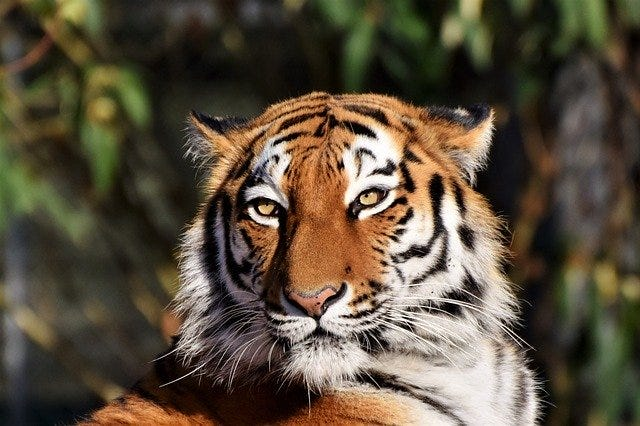# Basic Image Classification in Tensorflow 2.0 (Part 1)

Source: Deep Learning on Medium# Basic Image Classification in Tensorflow 2.0 (Part 1)

Training an Image Classification model that classifies your data-set has become a very easy task with Tensorflow and Keras. Before we train our Image Classifier, I would like to answer a couple of basic questions.

# What is Image Classification?

Image classification refers to a process in computer vision that can classify an image according to its visual content. For example, an Image Classification model/algorithm may be designed to tell if the input image contains tiger or not.

# How is Image Classification different from Object Detection?

Image Classification just outputs one class label that has the highest probability, whereas Object Detection outputs all the class labels with probability higher than threshold probability in the image along with their respective bounding boxes.

# Types of Image Classifications:

1. Binary Image Classification (Two classes only)
2. Multi-class Image Classification (Two or more classes)

# Workflow

Our workflow for building an Image Classification model is as follows

2. Build the model
3. Train the model
4. Test the model
5. Improve and repeat if required

# Binary Image Classifier

Okay, now we will train a Binary Image Classifier that classifies the given image as either elephant or tiger. Lets start with importing tensorflow.

`import tensorflow as tf`

Our Image Classification model will be a Sequential model with a number of Dense and Convolution layers. NumPy is used to convert python list to numpy array and to perform required matrix operations and `matplotlib.pyplot` is required to plot graphs and display images. Let’s import the following packages.

`from tensorflow.keras.models import Sequentialfrom tensorflow.keras.layers import Dense, Conv2D, Flatten, Dropout, MaxPooling2Dfrom tensorflow.keras.preprocessing.image import ImageDataGeneratorimport osimport numpy as npimport matplotlib.pyplot as plt`

`ImageDataGenerator` class makes it easier to load our data. But before loading our data, we need to structure our dataset in the following directory structure.

`project_directory|__ train |______ elephants: [indian_elephant.jpg, img001.jpg ....] |______ tigers: [tiger.jpg, tiger_white.jpg, img112.jpg ...]|__ validation |______ elephants: [elephants01.jpg, african_elephant.jpg ...] |______ tigers: [tiger_a.jpg, img201.jpg, img002.jpg ...]`

Let’s assign variables `train_dir` and `validation_dir` holding the proper file path for the training and validation set.

`train_dir = 'train'validation_dir = 'validation'`

# Analyze Data (Optional)

Let’s assign variables for training and validation set sub directories.

`train_elephants_dir = os.path.join(train_dir, 'elephants') # directory with our training elephant picturestrain_tigers_dir = os.path.join(train_dir, 'tigers') # directory with our training tiger picturesvalidation_elephants_dir = os.path.join(validation_dir, 'elephants') # directory with our validation elephant picturesvalidation_tigers_dir = os.path.join(validation_dir, 'tigers') # directory with our validation tiger pictures`

Let’s look at how many elephants and tiger images are in the training and validation directory.

`num_elephants_tr = len(os.listdir(train_elephants_dir))num_tigers_tr = len(os.listdir(train_tigers_dir))num_elephants_val = len(os.listdir(validation_elephants_dir))num_tigers_val = len(os.listdir(validation_tigers_dir))total_train = num_elephants_tr + num_tigers_trtotal_val = num_elephants_val + num_tigers_valprint('total training elephant images:', num_elephants_tr)print('total training tiger images:', num_tigers_tr)print('total validation elephant images:', num_elephants_val)print('total validation tiger images:', num_tigers_val)print("--")print("Total training images:", total_train)print("Total validation images:", total_val)`

Let’s set up few variables to use while pre-processing the dataset and training the network. Let’s resize all the images into 150x150.

`batch_size = 128epochs = 15IMG_HEIGHT = 150IMG_WIDTH = 150`

`ImageDataGenerator` class helps us pre-process the dataset images and convert them into batches of tensors.

`train_image_generator = ImageDataGenerator(rescale=1./255) # Generator for our training datavalidation_image_generator = ImageDataGenerator(rescale=1./255) # Generator for our validation data`

`flow_from_directory` method load images from the disk, applies rescaling, and resizes the images into the required dimensions. We choose `class_mode='binary'` as our model is a Binary Image Classification model.

`train_data_gen = train_image_generator.flow_from_directory(batch_size=batch_size, directory=train_dir, shuffle=True, target_size=(IMG_HEIGHT, IMG_WIDTH), class_mode='binary')val_data_gen = validation_image_generator.flow_from_directory(batch_size=batch_size, directory=validation_dir, target_size=(IMG_HEIGHT, IMG_WIDTH), class_mode='binary')`

# Build the model

Let’s build a model with three convolution blocks with a max pool layer in each of them. There’s a fully connected (Dense) layer with 512 units on top of it that is activated by a `relu` activation function. The model outputs class probabilities based on binary classification by the `sigmoid` activation function.

`model = Sequential([ Conv2D(16, 3, padding='same', activation='relu', input_shape=(IMG_HEIGHT, IMG_WIDTH ,3)), MaxPooling2D(), Conv2D(32, 3, padding='same', activation='relu'), MaxPooling2D(), Conv2D(64, 3, padding='same', activation='relu'), MaxPooling2D(), Flatten(), Dense(512, activation='relu'), Dense(1, activation='sigmoid')])`

# Compile the model

Let’s choose the ADAM optimizer and binary cross entropy loss function for our model. To view training and validation accuracy for each training epoch, pass the `metrics` argument.

`model.compile(optimizer='adam', loss='binary_crossentropy', metrics=['accuracy'])`

# Model summary

`summary` method is used to view all the layers of the network.

`model.summary()`

# Train the model

Use the `fit_generator` method of the `ImageDataGenerator` class to train the network. Training the model may take few minutes to several hours depending on the size of the dataset.

`history = model.fit_generator( train_data_gen, steps_per_epoch=total_train // batch_size, epochs=epochs, validation_data=val_data_gen, validation_steps=total_val // batch_size)`

# Visualize training results

Let’s visualize the results after the completion of the training.

`acc = history.history['accuracy']val_acc = history.history['val_accuracy']loss = history.history['loss']val_loss = history.history['val_loss']epochs_range = range(epochs)plt.figure(figsize=(8, 8))plt.subplot(1, 2, 1)plt.plot(epochs_range, acc, label='Training Accuracy')plt.plot(epochs_range, val_acc, label='Validation Accuracy')plt.legend(loc='lower right')plt.title('Training and Validation Accuracy')plt.subplot(1, 2, 2)plt.plot(epochs_range, loss, label='Training Loss')plt.plot(epochs_range, val_loss, label='Validation Loss')plt.legend(loc='upper right')plt.title('Training and Validation Loss')plt.show()`

You can find the entire code here. In upcoming parts, we shall look how to improve models performance and build Multi-class Image Classification models.